# LEAST LAXITY FIRST (LLF) SCHEDULING ALGORITHM

Least Laxity First (LLF) is a job level dynamic priority scheduling algorithm. It means that every instant is a scheduling event because laxity of each task changes on every instant of time. A task which has least laxity at an instant, it will have higher priority than others at this instant.

### Introduction to Least Laxity first (LLF) scheduling Algorithm

More formally, priority of a task is inversely proportional to its run time laxity.  As the laxity of a task is defined as its urgency to execute. Mathematically it is described as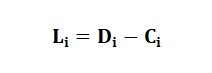Here di is the deadline of a task, Ci is the worst-case execution time(WCET) and Li is laxity of a task. It means laxity is the time remaining after completing the WCET before the deadline arrives. For finding the laxity of a task in run time current instant of time also included in the above formula.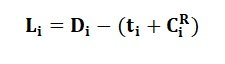Here  is the current instant of time and  is the remaining WCET of the task. By using the above equation laxity of each task is calculated at every instant of time, then the priority is assigned. One important thing to note is that laxity of a running task does not changes it remains same whereas the laxity all other tasks is decreased by one after every one-time unit.

### Example of Least Laxity first scheduling Algorithm

An example of LLF is given below for a task set.

 Task Release time(ri) Execution Time(Ci) Deadline (Di) Period(Ti) T1 0 2 6 6 T2 0 2 8 8 T3 0 3 10 10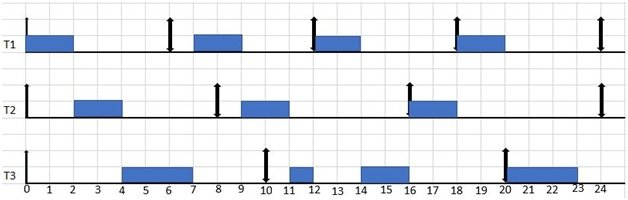Figure 4. LLF scheduling algorithm

1. At t=0 laxities of each task are calculated by using equation 4.2. as

L1   = 6-(0+2) =4

L2 = 8-(0+2) =6

L3= 10-(0+3) =7

As task T1 has least laxity so it will execute with higher priority. Similarly, At t=1 its priority is calculated it is 4 and T2 has 5 and T3 has 6, so again due to least laxity T1 continue to execute.

1. At t=2 T1is out of the system so Now we compare the laxities of T2 and T3 as following

L2= 8-(2+2) =4

L3= 10-(2+3) =5

Clearly T2 starts execution due to less laxity than T3. At t=3 T2 has laxity 4 and T3 also has laxity 4, so ties are broken randomly so we continue to execute T2. At t=4 no task is remained in the system except T3 so it executes till t=6. At t=6 T1 comes in the system so laxities are calculated again

L1 = 6-(0+2) =4

L3= 10-(6+1) =3

So T3 continues its execution.

1. At t=8 T2 comes in the system where as T1 is running task. So at this instant laxities are calculated

L1  = 12-(8+1) =3

L2= 16-(8+2) =6

So T1 completes its execution. After that T2 starts running and at t=10 due to laxity comparison T2 has higher priority than T3 so it completes it execution.

L2= 16-(10+1) =5

L3= 20-(10+3) =7

t=11 only T3 in the system so it starts its execution.

1. At t=12 T1 comes in the system and due to laxity comparison at t=12 T1 wins the priority and starts its execution by preempting T3. T1 completes it execution and after that at t=14 due to alone task T3 starts running its remaining part. So, in this way this task set executes under LLF algorithm.

LLF is an optimal algorithm because if a task set will pass utilization test then it is surely schedulable by LLF. Another advantage of LLF is that it some advance knowledge about which task going to miss its deadline. On other hand it also has some disadvantages as well one is its enormous computation demand as each time instant is a scheduling event. It gives poor performance when more than one task has least laxity.

### Enhanced Least Laxity First Scheduling Algorithm

LLF is good for avoiding transient overload and domino effect occurring in EDF. But it also has a shortcoming, that is thrashing. When more than one task has least laxity than this phenomenon of Thrashing occurs. Thrashing is the behavior of preemption by the tasks one another at each time tick. This kind of preemption results in context switch at each instant of time. This context switching results in more computation power consumption as switching from one task to another which result in saving and loading registers, memory mapping and updating many tables and lists. So, it results in loss of computation time as well. To overcome this short come of LLF an improved version of LLF is introduced that is Enhanced least laxity first scheduling algorithm (ELLF). IN ELLF whenever more than one task has least laxity than they all are grouped together and EDF is applied within the group whereas taking this group as a single task LLF is applied to this group and other remaining tasks in the task set.

An example of LLF with thrashing is given below

 Task Release time(ri) Execution Time(Ci) Deadline (Di) Period(Ti) T1 0 3 12 7 T2 0 4 13 10 T3 0 5 14 12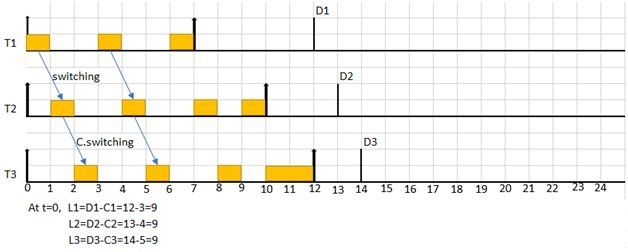So, to overcome this context switching due to thrashing We employ ELLF on the same example by grouping together the tasks having same least laxity, then apply EDF to avoid context switching.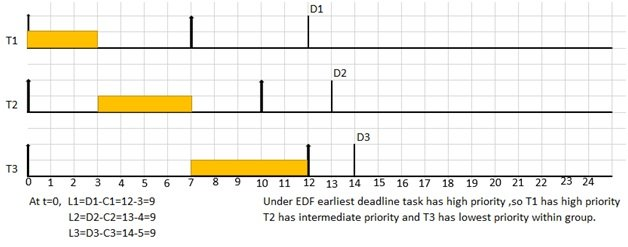So this is all about enhanced least laxity first scheduling Algorithm. You may also like to read:

### 3 thoughts on “LEAST LAXITY FIRST (LLF) SCHEDULING ALGORITHM”

1. Hi there. thank you for your efforts in making an example about LLF algorithm, but I have two questions about the LLF example. 1st: at t=6 why you calculated L1 with t=0?. 2nd: if we applied what happens in my 1st question on T1 again in t=12 that means L1=12-(0+2) =10 and then L3=20-(12+2)=6 and in this case T3 will have the least laxity than T1, so T3 should be start its execution, am I right?